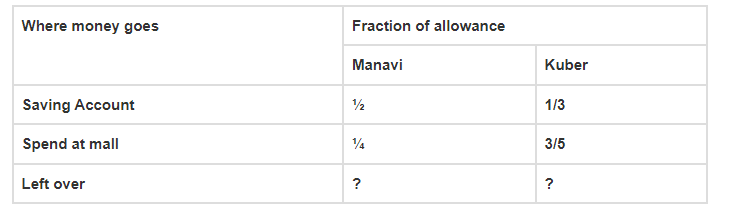# Manavi and Kuber each receives an equal allowance.

Question:

Manavi and Kuber each receives an equal allowance. The table shows the fraction of their allowance each deposits into his/her saving account and the fraction each spends at the mall. If allowance of each is ₹ 1260 find the amount left with each.

Solution:From the question,

Manavi and Kuber each receives and equal allowance = ₹ 1260

Let us assume total cost be ₹ 1

For Manavi, left over = Total cost – Total spends

= 1 – (½ + ¼)

= 1 – (2 + 1)/4

= 1 – (3/4)

= (4 – 3)/4

= ¼

So, Amount = 1260 × ¼ = ₹ 315

For Kuber, left over = Total cost – Total spends

= 1 – (1/3 + 3/5)

= 1 – (5 + 9)/15

= 1 – (14/15)

= (15 – 14)/15

= 1/15

So, Amount = 1260 × (1/15) = ₹ 84